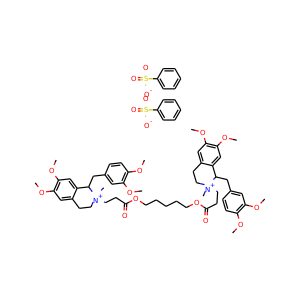877-202-0205     support@chemchart.com     @chemchart

Sign In    RegisterCisatracurium besylate
Weight 1243.501 g/mol C65H82N2O18S2 18 0 6 26

# Cisatracurium besylate (96946-42-8)

"What do you need help with?"### Alternate Names

• SMILES
C[N+]1(CCC2=CC(=C(C=C2C1CC3=CC(=C(C=C3)OC)OC)OC)OC)CCC(=O)OCCCCCOC(=O)CC[N+]4(CCC5=CC(=C(C=C5C4CC6=CC(=C(C=C6)OC)OC)OC)OC)C.C1=CC=C(C=C1)S(=O)(=O)[O-].C1=CC=C(C=C1)S(=O)(=O)[O-]
• InChIKey
XXZSQOVSEBAPGS-UHFFFAOYSA-L